# Math in Focus Grade 4 Chapter 7 Practice 5 Answer Key Rounding Decimals

Practice the problems of Math in Focus Grade 4 Workbook Answer Key Chapter 7 Practice 5 Rounding Decimals to score better marks in the exam.

## Math in Focus Grade 4 Chapter 7 Practice 5 Answer Key Rounding Decimals

Fill in the missing number in each box.
Then round each decimal to the nearest whole number.

Example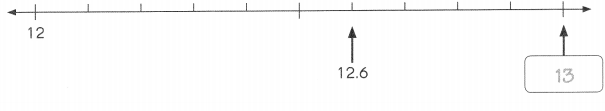12.6 rounded to the nearest whole number is 13.

Question 1.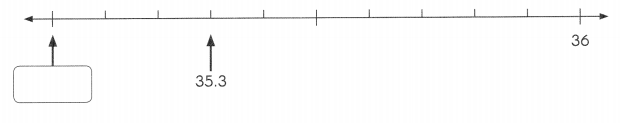35.3 rounded to the nearest whole number is ____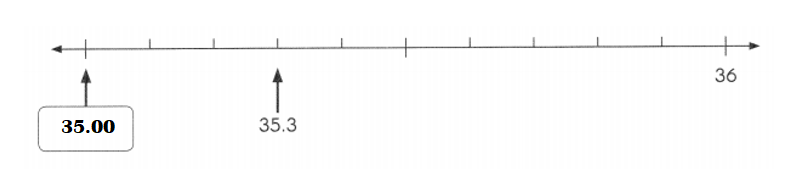Explanation:
35.3 rounded to the nearest whole number is 35.00.

Question 2.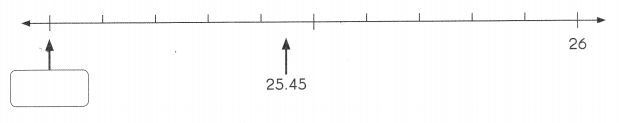25.45 rounded to the nearest whole number is ___________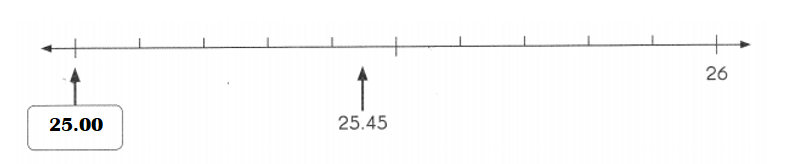Explanation:
Nearest whole number of 25.45 = 25.00.

Question 3.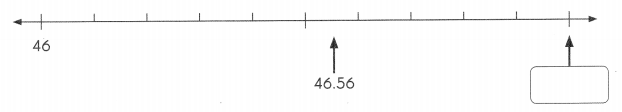46.56 rounded to the nearest whole number is ___.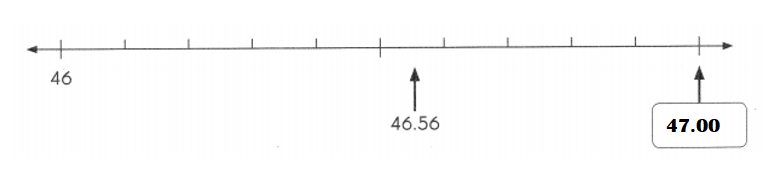Explanation:
46.56 rounded to the nearest whole number is 47.00.

Round each measure.
Question 4.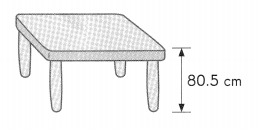Round the height of the table to the nearest centimeter.
____________ centimeters is about ____________ centimeters.
Height of the table to the nearest centimeter = 81.00 cm.
80.5 centimeters is about 81.00 centimeters.

Explanation:
Height of the table = 80.5 cm.
Height of the table to the nearest centimeter = 81.00 cm.
80.5 centimeters is about 81.00 centimeters.Question 5.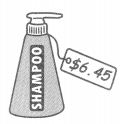Round the price of the shampoo to the nearest dollar.
$___ is about$ ____.
Price of the shampoo to the nearest dollar = $6.00.$6.45 is about $6.00. Explanation: Price of the shampoo =$6.45.
Price of the shampoo to the nearest dollar = $6.00.$6.45 is about \$6.00.

Question 6.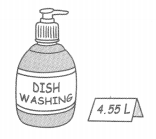Round the amount of detergent to the nearest liter.
____ liters is about ___ liters.
Amount of detergent to the nearest liter = 5.00.
4.55 liters is about 5.00 liters.

Explanation:
Amount of the detergent = 4.55 L.
Amount of detergent to the nearest liter = 5.00.
4.55 liters is about 5.00 liters.Question 7.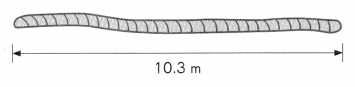Round the length of the rope to the nearest meter.
___________ meters is about ___________ meters.